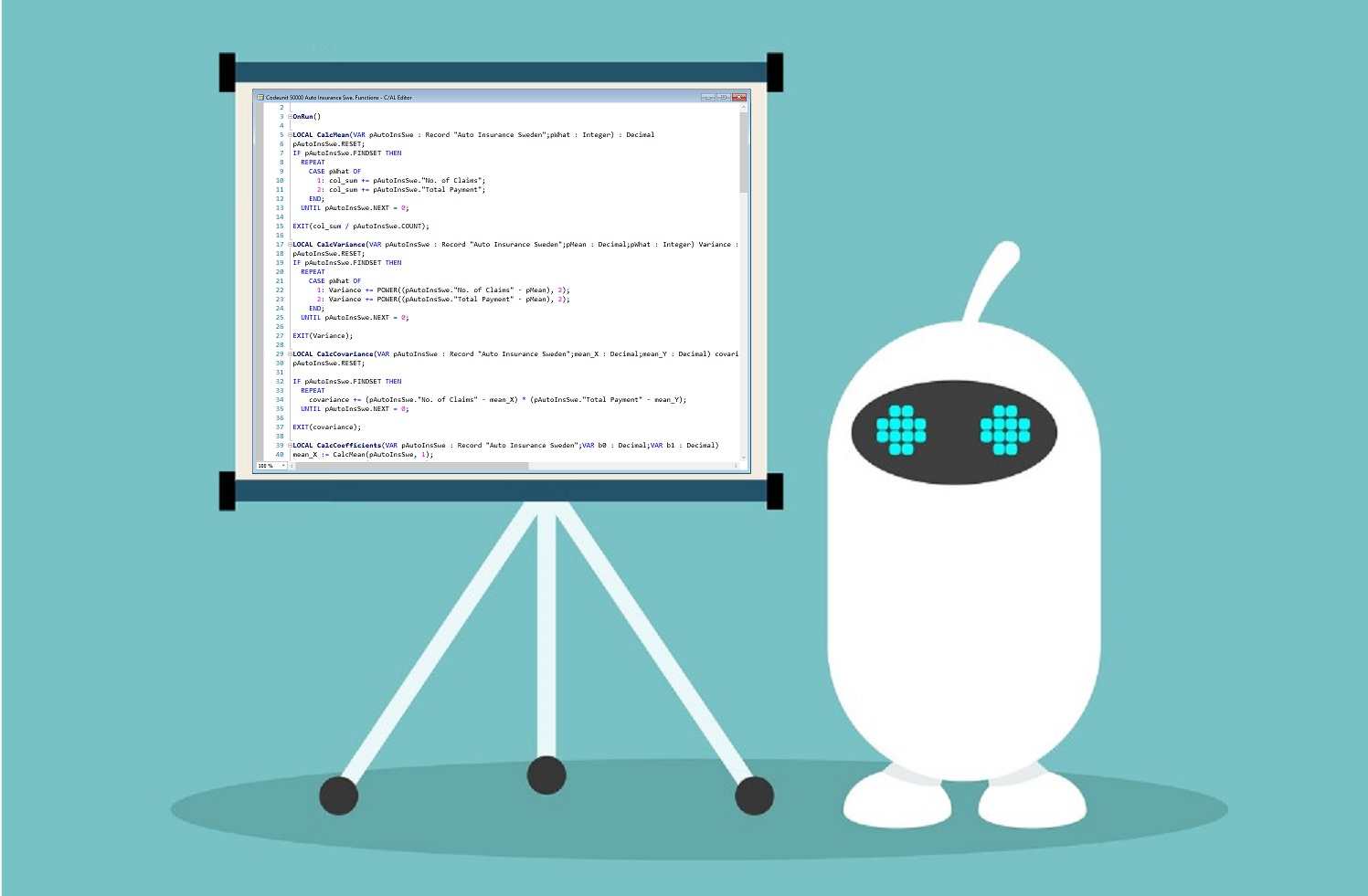Machine Learning from scratch with C/AL !

Microsoft announced at Directions ASIA that C/AL will be ceased in the next major release in autumn 2019. To celebrate the final days of C/AL, I’ve set myself the challenge to realize a Machine Learning Model within C/AL. And yes, it was possible!

But, I have to be fair. Using this code, NAV will not start to speak or de-install itself. And even it would be every accountant’s dream, my model will not enable you to process image or speech recognition through your good old NAV-client. I’ve orientated myself at a typical Machine Learning problem which students learn in their early days. It’s easy and understandable. You can download the objects here. Let’s jump straight in…

### The problem

My implementation is a Simple Linear Regression algorithm for the Swedish Auto Insurance data set. The data set contains a X and Y column. The X column represents the number of claims and the Y represents the total payments in Swedish Kronor. The idea is, that the more claims do exists, the more money was paid.

Using Simple Linear Regression it is possible to identify the relation between the number of claims and the total payment. This relation can be stored and used for, to predict the total payment for a new number of claims. In reality, this could be used to estimate a budget for a new period. For example, the insurance company expects 100 claims in the new period, how much money do they have to put aside?

### Simple Linear Regression

The below picture demonstrates how the Simple Linear Regression works. The blue elements are from the source data. The red boxes are the calculated predictions. The Simple Linear Regression calculates where to draw the line between all the blue elements, so that the difference to the red boxes is minimal as possible.

Simple Linear Regression is more than 200 years old and well understood. It’s good start for beginner and it is possible to implement it using C/AL.

My implementation is seperated into 4 objects. The below list provides a description and an overview of the details

• Table 50000 “Auto Insurance Sweden”
• This is the table which provides the training data.
• Because the Auto Insurance Sweden dataset contains 2 columns, this table provides two columns to import data into (“No. of Claims” which is X in the source file and “Total Payment” which is Y in the source file).
• On top of this, the table contains the field “Index” which is populated in the OnInsert-Trigger automatically and used as primary key.
• XMLport 50000 “AutoInsSwe Import/Export”
• This the XMLport to import the csv-files. The package contains two files: a modified version of the Auto Insurance Sweden dataset and a simple dataset for easier understanding
• Codeunit 50000 “Auto Insurance Swe. Functions”
• The codeunit is the heart of the implementation and provides the following necessary functions.
• CalcMean
• This function calculates the mean value for all values of either the “No. of Claims” or the “Total Payment” field.
• CalcVariance
• This function provides the variance of all values for both fields. The variance represent information how far all the numbers reach out from the mean.
• The following picture shows a visual explanation what the variance is.
• CalcCovariance
• The covariance represents information about how two values are correlated to each other. For example, when the first values doubles, will the second value double too?
• CalcCoefficients
• Refering to the picture above, the coefficients determine where the prediction-line starts and with which angle it is drawn.
• This function delivers the integral information to predict new values.
• To calculate the coefficients, these two formulas are used. This explains also the need for the other functions.
• CalcPredictions
• This function calls the function “CalcCoefficients” with the training dataset. That means, the training dataset is analysed and the coefficients are determined.
• The coefficients of the training dataset are used to calc a prediction on the test dataset.
• Calc_RMSE_metric
• Thsi function sums up the difference between the prediction and the actual value.
• This number tells you the performance of the model.
• RMSE is an abbrevation for Root Mean Square Error.
• train_test_split
• This function is able to split a given dataset into a training and test dataset.
• The function takes a parameter for the split between training and test.
• If a record will be assigned to the training or test dataset is randomized.
• Learn_Evaluate_Algorithm
• This function is used to execute the model-training.
• GetCoefficients
• It is necessary to return the coefficients (two values) to the page.
• Predict
• Predicting a new value is done in this function.
• It is necessary to provide the new value and the coefficients from the training as parameters.
• Page 50000 “Auto Insurance Swe ML”
• The graphical user interface is simply and functionald designed.
• Start from the left to the right:
• Click “Import/Export” to import the training dataset
• When data is available, click “Evaluate” which calculates the coefficients.
• After this you can enter “Expected No. of Claims” and click “Predict”.